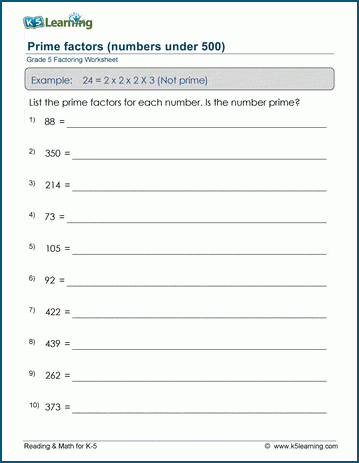# Prime factors (< 500)

## Math worksheets: Prime factors of numbers under 500

Below are six versions of our grade 5 math worksheet on prime factors. Students must determine the set of prime numbers which multiply together to give the original number. A prime number can only be divided evenly  only by 1 and itself.  These worksheets are pdf files.## More factoring & prime factors worksheets

Explore all of our factoring worksheets, from factoring numbers under 50 to prime factor trees, GCFs and LCMs.

What is K5?

K5 Learning offers free worksheets, flashcards and inexpensive workbooks for kids in kindergarten to grade 5.  We help your children build good study habits and excel in school.